## jueves, 9 de febrero de 2012

### The Rayleigh-Ritz method explained for humans

Preliminaries
At last, I feel ready to explain the key concept needed to follow the steps in the Finite Element Method: the notion of STRAIN ENERGY!
The problem with this concept is that is as simple as it looks, ergo all the attached complications must come from somewhere else...
For the animation below I have chosen a classic spring to illustrate the concept (yes I know, is also a classic example...). Could have been anything else capable of storing energy, as a phone battery or a dam, but this seems more adequate given we are talking about structures.
The graph (click on it to see it moving) represents the relation between the applied force to stretch the spring and the actual displacement of the spring. So far, this is pure Hooke's law from secondary school (F=Kx)...
Also, the area that gets shadowed when the spring gets "tensioned" is, in mathematical terms, an integral of the drafted curve.Displacement e vs Force F on a spring of elastic material. Shaded area represents stored strain energy. When pulled with a force F its tip deforms an amount e. When released it pulls back with the same force F.
Given this particular, although very common case of a material (an elastic one), there is a straight tilted part in the curve that is called "elastic domain". If one keeps pulling the spring further then we still wouldn't break it, but instead we cannot expect it to recover its initial shape. The spring has plastified and we are in the horizontal part of the graph...but that is another story.
So, this "triangle" underneath the tilted part has an area, an integral, a scalar value in squared centimeters. The value of such integral is, not in centimeters but in N per meter or Joules (we are comparing force vs displacement), so is an Energy!Applied force on the tip F vs potential energyDisplacement e vs potential energy
And the shape of its curve, against the same displacement e or force F (click the animations) happens to be that of a parable (wasn't it obvious?)...
Well, and so far for the basics. Let's now think of a solid beam or column and put it in the place of our spring. When loaded, it would deform, would store elastic energy in its material ready to be released as soon as the applied force leaves. Normally things tend not to greedily accumulate energy but just the opposite. In Nature, a state of balance is that where things store a minimum amount of potential energy.
This basic principle in general applies for almost everything in life, and always does in physics. A drop of water in a cloud that has absorbed thermal energy and stored some of it as potential energy would release this as soon as it can, becoming rain when there is a lot of them, a capacitor in a circuit or even the adapter of a laptop store a differential of potential that make them useful, etc. This is the famous principle of minimum total potential energy (not to be mistaken with the principle of minimum energy, which also applies but in other fields).

Relating strain energies and forces

The way engineers have found a beam or column or piece of metal can store energy is by means of five types of loading: axial, bending, torsion, direct shear and traverse shear. Actually this is the way they have found they can stress things. As for every stress there should be associated a strain and hence some potential energy, this is tantamount as to say that they store energy. Though disputable, this is a convention and so we will accept it in the absence of a better one.Resume of the strain energies associated to each type of applied force in engineering. Traverse shear has been omitted as is generally neglected.
The good thing about this convention is that the job of assimilating stored potential energies for each type has already been done for us and put up in the shape of a formula. The table above gives the relation for each one of them in relation to the applied force and in relation to the caused strain.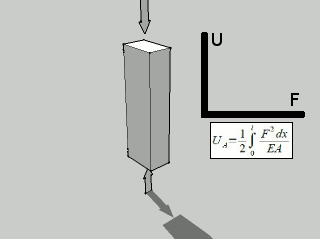The compressed beam under axial force F stores energy U of a value according to the formula.The same beam, under flexural bending moment.Similarly for shear...and now for torsion
The Rayleigh-Ritz method

And now we have all the concepts and are ready to work.
The Rayleigh–Ritz method (after Walther Ritz and Lord Rayleigh-Wikipedia), is considered a variational method. This is so because it is based in the calculus of variations. When applied to structural mechanics the varying value is the value of the strain potential energy, that is to be equilibrated against the work done by the external forces.
What we will try to do is to find what is the configuration, the state of the beam or group of beams, where the total energy of the system "external forces + internal stresses" gives a lowest value for the value of the energy.

It has eight fairly simple steps (this is also arguable, but this is how I understand it best) which I will try to picture as clearly as possible:

Step 1: Define the internal stored energy function (always an integral from the table above or a combination of them) and the external (a function of the caused displacement times the applied force).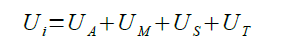The internal strain energy is the sum of all the possible combinations of internal strains from the table above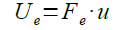The external applied energy is the result of multiplying the external forces by the displacement

Step 2: Arbitrarily and happily (well, almost...) choose one function where the dimensions of the beam and the variable in the internal stored energy function get related. Multiply it by a dummy parameter (we will call it u overlined). The function chosen v is an approximation, so we overline it also to denote this.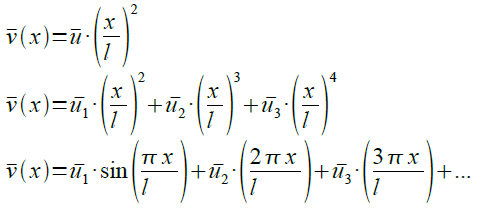The "dummy function" we will introduce into the energy formula can be any from above. The more elaborated, the higher the precision.Two possibilities of approximation functions (without the U parameter). It is known that beams deflect quadratically.

Step 3: Solve, by means of integral calculus, the integral in step 1 with the chosen function in step 2. This will provide a relation between the dummy parameter and the internal energy. For the example below, where normally only bending moment is considered: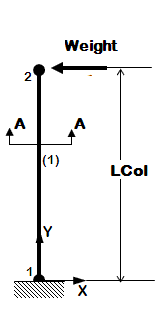Laterally loaded beam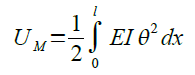Internal strain energy due to the weight on the tip is given here as a function of the angle of rotation of the beam, but also is generally rounded as the second derivative of the displacement (v'').Obtention of the second derivative of the "dummy" function

A very important remark: it is assumed from the beginning that the result will not be accurate!!

Step 4: Do the same for the external applied energy.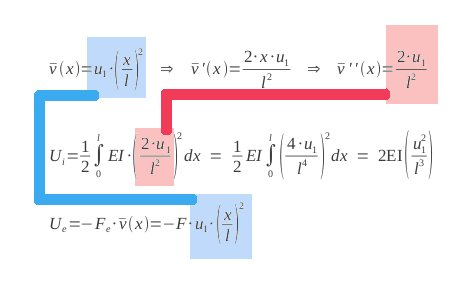The "dummy" function and its second derivative substituted into the potential strain (Ui) and the external (Ue) energies.
Step 5: Add the two energies in order to get the total energy of the system, V (column internal + external forces)

Step 6: Applying the theorem of minimum total potential energy, the derivative of the total energy with respect to the parameter must be 0.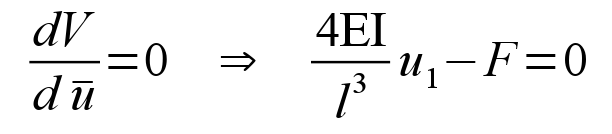Step 7: Get the value of the parameter from the equation above.

Step 8: Substitute its value in the step 2 to get the real function of the displacement.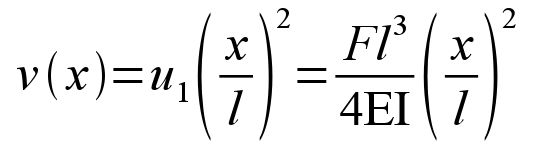And that is it. For whatever value of x along the beam now we have a value of the deflection. Unfortunately the approximation chosen v(x)=u1(x/l)^2 is not very accurate, so a calculated displacement of the tip would diverge at least 25% from the analytical...but that is another story...
The most difficult steps are actually 1 and 2, where one should memorize the functions in order to master the method. The rest is easy algebra and very simple first order derivatives.
The Rayleigh-Ritz method eventually evolved into the nowadays ubiquitous Finite Element (did you recognize the shape function there?).

Well, I really hope this helps anyone who reads it as much as it helped me to write it...

Enjoy!

#### 6 comentarios:

1.I have just discover you site about a month ago and I really do like what you are doing with sharing all these. Keep it going. :)

2.Shape function is step 8 ??
In a spring U=Ke^2 . But K*e=F , So U=F*e . So U/e = F ?? Shouldnt that be linear ??

U : Strain energy.
e : Displacement.
F : Pulling Force.

1.Hi Pruthvi,
Shape function is chosen in step 2. Step 8 is the resulting horizontal displacement calculated on the basis of that particular shape function. If a different shape function had been chosen in step 2, a different equation would have arisen out of Rayleigh-Ritz method.
Regarding the formulas, notice that also e=F/K, hence U=F*F/K=F^2/K, which is quadratic.
However, as you pointed out well, hey are linear when e and F are independent. But then the value of K doesn't apply anymore and we are in the plastic range. I suggest you to make a very simple Excel exercise where e, F and U are pictured according to the Strain-stress curve to realize this.

Thanks again for commenting! :)

3.Dude, thanks a lot for your explanations. My teacher sucked at teaching this, and the available literature is indeed not for humans, it seems to be destined at people that already know these things. It's very hard to get a mental picture of stuff, when it's pages after pages of rambling about mathematical formulas and derivations, without getting to the "why is it done this way, and not another". Thanks a lot for your insights again.

4.Thanks a lot it really helped.

5.I found a solution for torsion strain using a first degree polynomial:
��(��)=(2 ���� ��^5 ������((�� ��)/��))/(��^6 �� ��)
Can I verify mathematically that my result is correct?
How could I assess the precision of the equation?
Thanks